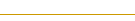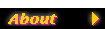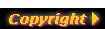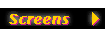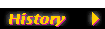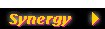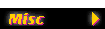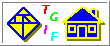William Chia-Wei Cheng (bill.cheng@usc.edu)## Tgif FAQ - Splines

Are the open spline objects tgif draws Bézier curves? If not, what are they?
Well, tgif doesn't really draw Bézier curves in general. If there are 3 control points or less, what tgif draws is a quadratic Bézier curve.

If there are n > 3 control points, tgif draws a curve which is composed of n-2 pieces of curves that, as a whole, is continuous, and has continuous first derivatives everywhere (with approximations). Since each piece has exactly 3 control points, each piece is drawn as a quadratic Bézier curve. So, I guess you can say that what tgif draws are piecewise quadratic Bézier curves.

Below is basically where the n-2 pieces come from for n > 3. I will ilustrate this with an example for n = 5.

First, change the line type to straight, you should see n-1 line segments connecting the n original control points as shows in Figure A.

Find the midpoints of all the middle n-3 line segments and the 2 endpoints of the original curve, as shown in Figure B. These are the endpoints for the quadratic Bézier curves.

This means that between any 2 consecutive points shown in Figure B, tgif draws a quadratic Bézier curve. Therefore, there are a total of n-2 quadratic Bézier curves. Please note that between any of these 2 consecutive points (let's call them P1 and P2), there's a vertex (let's call it P3) which is a control point of the original tgif spline object. From 3 control points, tgif already knows how to draw a quadratic Bézier curve (see the top of this page). Tgif just uses the same algorithm to draw the n-2 quadratic Bézier curves. Below is a more detailed algorithm tgif uses for drawing (approximating) a quadratic Bézier curve having control points P1, P3, and P2.

The basic algorithm for drawing a quadratic Bézier curve from control points P1, P3, and P2 is depicted in Figure C below. The quadratic Bézier curve is shown in red. Please note that the curve is tangent to line segment P1-P3 at P1 and it is also tangent to line segment P2-P3 at P2.

P4 is the midpoint of line segment P1-P3 and P5 is the midpoint of line segment P2-P3. Furthermore, P6 is the midpoint of line segment P4-P5. The algorithm recursively draws two quadratic Bézier curves, one having control points P1, P4, and P6 and one having control points P2, P5, and P6. Clearly, the curve and the first derivative of the curve are continuous at point P6. Using this algorithm for Figure B, the 3 quadratic Bézier curve are shown in Figures D, E, and F.

Overlaying Figures D, E, and F together, the result is shown in Figure G below.